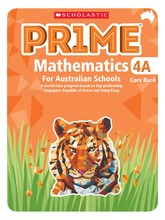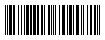# Prime Aus Mathematics Sb 4 A Scholastic## Stock information

### General Fields

• : \$17.00(AUD)
• :
• : 9789814709071
• : Scholastic Education Australia
• : Scholastic Education Australia
• :
• :
• :
• :
• :
• : 17.0
• :
• :
• :
• : books

### Special Fields

• :
• :
• :
• : Prime Maths
• :
• :
• :
• :
• :
• :
• :
• :
• :
• :
• :
• :9789814709071

## Description

###### Chapter 5: Angles

Lesson 1: Angle Measures

Lesson 2: Turns and 8-point Compass

Lesson 3: Problem Solving

###### Chapter 6: Perpendicular and Parallel Line Segments

Lesson 1: Drawing Perpendicular Line Segments

Lesson 2: Drawing Parallel Line Segments

###### Chapter 7: Squares and Rectangles

Lesson 1: Properties of Squares and Rectangles

###### Chapter 8: Area and Perimeter

Lesson 1: Perimeter

Lesson 2: Area of a Rectangle

Lesson 3: Squares and Rectangles

Lesson 4: Composite Figures

Lesson 5: Problem Solving

###### Chapter 1: Whole Numbers

Lesson 1: Numbers to 100 000

Lesson 2: Rounding Numbers

Lesson 3: Factors

Lesson 4: Multiples

###### Chapter 2: Multiplication and Division of Whole Numbers

Lesson 1: Multiplication by 1-digit Numbers
and by 10

Lesson 2: Division by 1-digit Numbers and by 10

Lesson 3: Multiplication by 2-digit Whole Numbers

Lesson 4: Problem Solving

View Sample Pages

###### Chapter 3: Fractions

Lesson 1: Mixed Numbers

Lesson 2: Improper Fractions

Lesson 4: Subtraction of Fractions

Lesson 5: Product of a Fraction and a Whole Number

Lesson 6: Conversion of Measurements

Lesson 7: Problem Solving

###### Chapter 4: Tables and Graphs

Lesson 1: Presenting Data

Lesson 2: Problem Solving